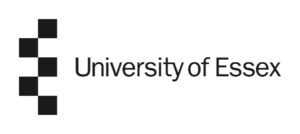The BSc (Hons) Mathematics with streams is an undergraduate degree offered in collaboration with the University of Essex.Mathematics is considered the language of science and technology, and is fundamental to many fields including Engineering, Economics, Computer Science, Physics, and Finance. As a result, there is a growing demand for individuals who possess strong mathematical skills and knowledge. The  BSc(Hons) Mathematics programme will provide students with a deep understanding of mathematical theory, along with practical skills in problem-solving, data analysis, and mathematical modelling.

The demand for mathematicians has been increasing worldwide with all projections showing this trend continuing for the foreseeable future. In the fields of Economics, Statistics and Computation, there is an increased need for experts with mathematical expertise. Additionally, there is a steady high demand for teachers of Mathematics, both in schools and in academia.

The 3 academic years comprises of core modules. In the 4th year, students chose among four streams:

• The programme is offered in collaboration with the University of Essex, ‘University of the Year‘ at the Times Higher Education Awards 2018.
• Essex is among the top 25 institutions in the UK for the quality of research.
• Essex is ranked 56th in the Times Higher Education Awards 2023 global rankings
• Modern lab.
• Aegean College is the only college in Greece offering a recognized degree in Mathematics.
• Opportunities for connecting students to the marketplace.
• The undergraduate program is offered in Athens and Thessaloniki.

The programme is consisted of 4 streams, Mathematics for Business, Mathematical Computation, Mathematics Education and Mathematical Statistics.

Students will be taught 8 modules of 15 credits each, which are common for all specialisations in year 1,2,3.

In year 4 students will be taught a 4 modules of 15 credits each in each specialisation followed by a 15 creits module titled Research in Mathematics as well as a 45 credits Dissertation. The total number of credits for the programme being 480 credits (240 ECTS).

Fall semester

• Fundamental Concepts of Mathematics.
• Calculus 1.
• Linear Algebra 1.
• Number Theory.

Spring semester

• Calculus 2.
• Linear Algebra 2.
• Introduction to Programming.
• Complex Analysis with Analytic Geometry.

Fall semester

• Differential Equations.
• Communicating Mathematics.
• Probabilities.
• Introduction to Computer Science.

Spring semester

• Real Analysis and Basic Topology.
• Statistics.
• Optimisation.
• Mathematical Modelling.

Fall semester

• Introduction to Economics.
• Introduction to Mathematics History, Philosophy and Education.
• Abstract Algebra.
• Applied Analysis.

Spring semester

• Differential Geometry.
• Introduction to Mathematical Physics.
• Numerical Methods.

Core Module

• Research Methods in Mathematics.
• Research Project in Mathematics.

• Econometrics.
• Microeconomics.
• Macroeconomics.
• Marketing and Management.

Mathematical Computation

• Discrete Mathematics.
• Graph Theory.
• Data Structures and Algorithms.

Mathematics Education

• Philosophy of Education.
• Psychology for Education.
• ICT in Mathematics Education.
• Teaching Mathematics.

Mathematical Statistics

• Game Theory.
• Stochastic Systems.
• Computational Statistics.

Aegean College provides modern facilities, computer labs, libraries and classrooms that meet the needs of students and academic staff.

In addition, students have access to the BioSensors SmartLab, an innovative artificial intelligence laboratory that features, among other software and tools, eye tracking and electroencephalogram (EEG) technology.

Graduates of the BSc (Hons) Mathematics can pursue a career in:

• Education.
• Research.
• Finance.
• Economics.
• Statistics.